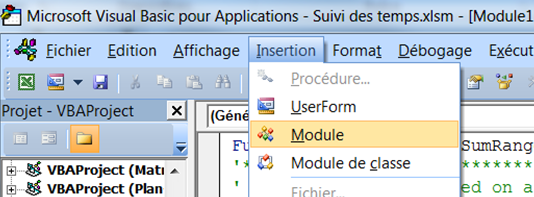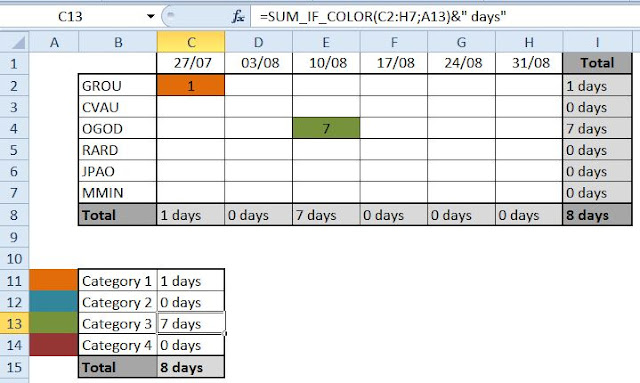## Wednesday, August 5, 2015

### Sum Excel cells based on the background color

Hi and let's talk about an easier subject, since everyone is on holydays!

For once, I'll not blog about Project but about Excel. Actually this is not the first time, I already blogged about the new timeline filtering features in Excel 2013.
Working on a timesheet report, I was looking for a way to sum the value of cells based on the background color. Indeed Excel reports are still extensively used with Project Server/Online and once the report is build, we often used formatting to make the report easier to read. So I knock at my colleague's door and beg for some help...

Note that strangely, what I'm going to explain below is not applicable for conditional formatting. Don't know why, but I suspect that the conditional formatting is not a "hard" background coloring and it would require to update the module but let's keep it simple for now and maybe this could feed a future post..

So basically, you have to start by creating a new module in Excel.
Press ALT F11, then insert, then module.Figure 1: new module creation in Excel

Then simply copy paste the following code, which is pretty simple to understand.

Function SUM_IF_COLOR(SumRange As Range, ColorRange As Range) As Variant
'*********************************************************
' Sum cells values based on a given color*
'*********************************************************
Application.Volatile
Dim Sum As DoubleDim Cel As Range

If ColorRange.Cells.Count > 1 Then
SUM_IF_COLOR = CVErr(xlErrValue)
Exit Function
End If
For Each Cel In SumRange
If Cel.Interior.ColorIndex = ColorRange.Interior.ColorIndex Then Sum = Sum + Cel
Next
SUM_IF_COLOR = Sum
End Function

Once done, you can create a new formula in your Excel spreadsheet like:
=SUM_IF_COLOR(Sum Range;Color)
Where :
• Sum Range is the range where the formula should look at the colored cells,
• Color is the cell with the color to detect.
Note also that the "application.volatile" allows the formula to be refreshed automatically when your range is updated in any ways.

Here is a simple example: my array is C2:H7 and the color to detect is A13. The cells C11 to C14 are automatically updated.Figure 2: example of the formula for summing values based on the background color

Et voilà!!

Do you have ideas for improving this formula? Or any workaround for the conditional formatting?

Share this article :